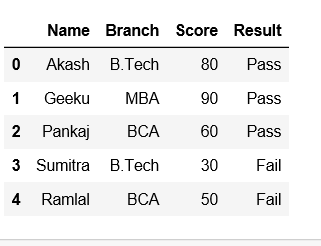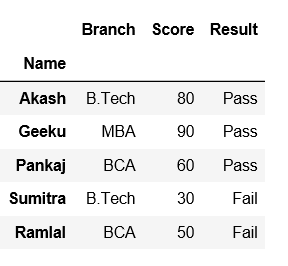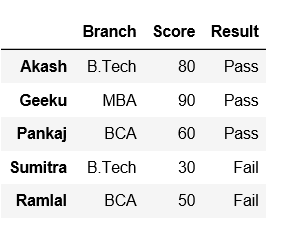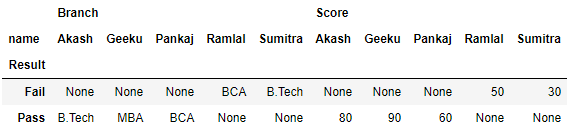# Convert a column to row name/index in Pandas

• Last Updated : 16 Jul, 2021

Pandas provide a convenient way to handle data and its transformation. Let’s see how can we convert a column to row name/index in Pandas.

Create a dataframe first with dict of lists.

## Python3

 `# importing pandas as pd``import` `pandas as pd` `# Creating a dict of lists``data ``=` `{``'Name'``:[``"Akash"``, ``"Geeku"``, ``"Pankaj"``, ``"Sumitra"``,``"Ramlal"``],``       ``'Branch'``:[``"B.Tech"``, ``"MBA"``, ``"BCA"``, ``"B.Tech"``, ``"BCA"``],``       ``'Score'``:[``"80"``,``"90"``,``"60"``, ``"30"``, ``"50"``],``       ``'Result'``: [``"Pass"``,``"Pass"``,``"Pass"``,``"Fail"``,``"Fail"``]}` `# creating a dataframe``df ``=` `pd.DataFrame(data)`` ` `df`

Output:Method #1: Using set_index() method.

## Python3

 `# importing pandas as pd``import` `pandas as pd` `# Creating a dict of lists``data ``=` `{``'Name'``:[``"Akash"``, ``"Geeku"``, ``"Pankaj"``, ``"Sumitra"``,``"Ramlal"``],``       ``'Branch'``:[``"B.Tech"``, ``"MBA"``, ``"BCA"``, ``"B.Tech"``, ``"BCA"``],``       ``'Score'``:[``"80"``,``"90"``,``"60"``, ``"30"``, ``"50"``],``       ``'Result'``: [``"Pass"``,``"Pass"``,``"Pass"``,``"Fail"``,``"Fail"``]}` `# Creating a dataframe``df ``=` `pd.DataFrame(data)` `# Using set_index() method on 'Name' column``df ``=` `df.set_index(``'Name'``)` `df`

Output:Now, set index name as None.

## Python3

 `# set the index to 'None' via its name property``df.index.names ``=` `[``None``]` `df`

Output:Method #2: Using pivot() method.
In order to convert a column to row name/index in dataframe, Pandas has a built-in function Pivot.
Now, let’s say we want Result to be the rows/index, and columns be name in our dataframe, to achieve this pandas has provided a method called Pivot. Let us see how it works,

## Python3

 `# importing pandas as pd``import` `pandas as pd` `# Creating a dict of lists``data ``=` `{``'name'``:[``"Akash"``, ``"Geeku"``, ``"Pankaj"``, ``"Sumitra"``, ``"Ramlal"``],``       ``'Branch'``:[``"B.Tech"``, ``"MBA"``, ``"BCA"``, ``"B.Tech"``, ``"BCA"``],``       ``'Score'``:[``"80"``, ``"90"``, ``"60"``, ``"30"``, ``"50"``],``       ``'Result'``: [``"Pass"``, ``"Pass"``, ``"Pass"``, ``"Fail"``, ``"Fail"``]}` `df ``=` `pd.DataFrame(data)` `# pivoting the dataframe``df.pivot(index ``=``'Result'``, columns ``=``'name'``)` `df`

Output:My Personal Notes arrow_drop_up